﻿ 3D surface with math animation online software ﻿ math handbook calculator - Fractional Calculus Computer Algebra System software+++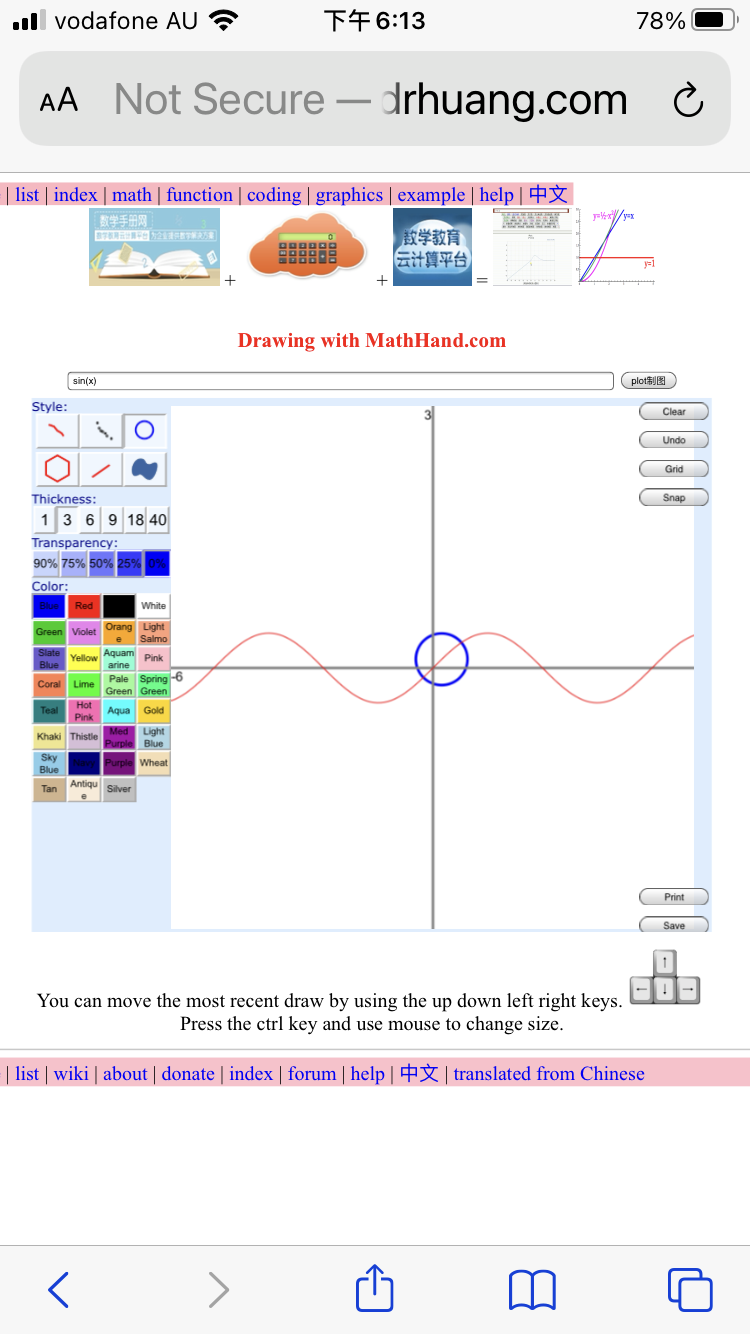=# Example of Math Graphics 数学函数图

## 3D surface

### surface3D(x)

1. surface3D
Please refresh this webpage to show different graphics, click the "Parametric Surfaces" to enlarge, click the "equation" to show 200 equations for graphics.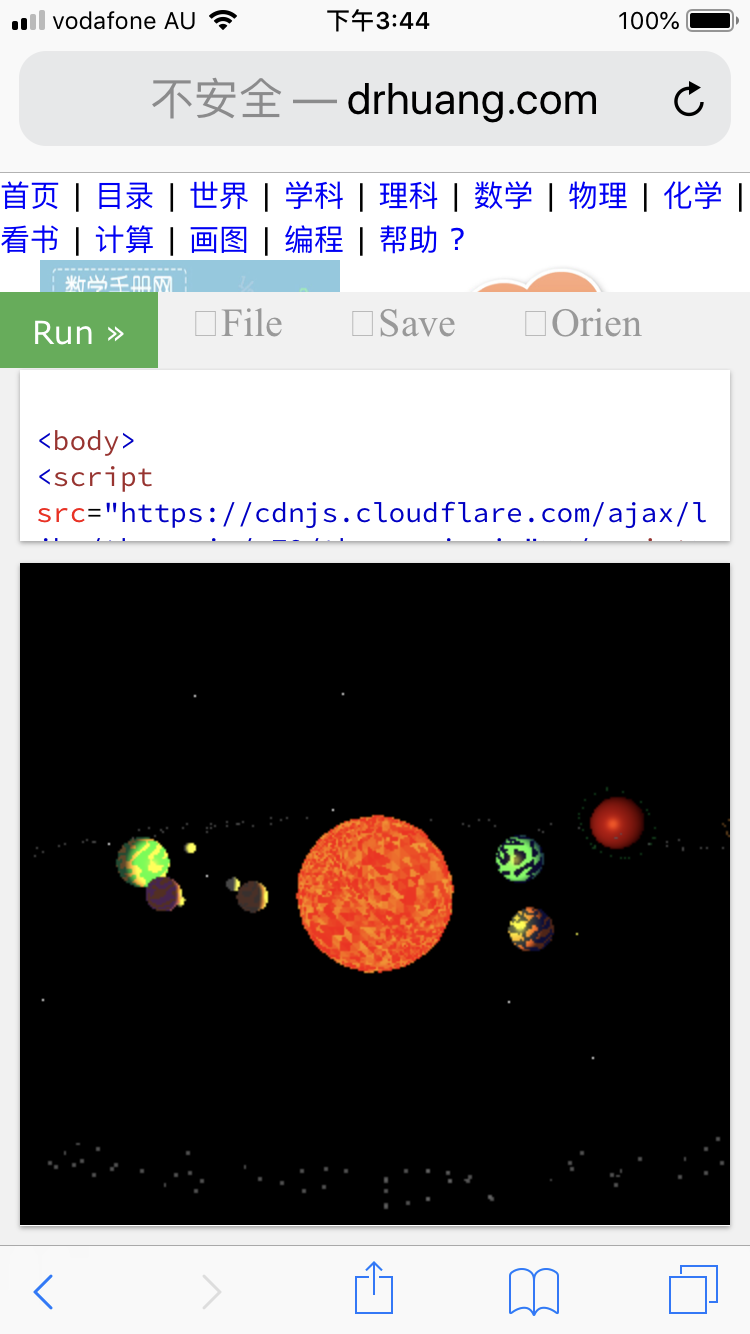solar 3D 互动太阳系 solar system 编辑 edit

2. solar 3D 互动太阳系
3. galaxy 3D 互动星系
4. bianchi-pinkall-animation
5. bianchi-pinkall-animation zoomed
6. Metaballs

### plot3DIE 制图3DIE 立体图IE

The plot3DIE( ) plots a function in 3D space by Internet Browser IE
7. plot3DIE( ) - plot3DIE(x*y) - plot3DIE(x*x, sin(x)) two surfaces overlap

The following plot functions did not work on Microsoft IE and QQ browsers. Please use Google Chrome browser.

plot3D(x*y), contour3D(x*y), wireframe3D(x*y), implicit3D(x*y*z), complex3D(x), parametric3D(t,t,t), parametric3Dxy(x,y,x*y), wireframe3Dxy(x,y,x*y), spin3D(x)
立体图, 立体外形图, 立体线框图, 立体隐函数图, 复数图, 立体参数图t, 立体参数图xy, 立体线框图xy, 旋转图3D

Notice that Microsoft Internet Explorer IE did not support svg, so IE cannot show following graph, please use other browrer.

### plot3D 制图3D

The plot3D( ) plots a function, implicit function, parametric function in 3D graph. The figure can be rotated by clicking and dragging with a mouse or swiping on a touch device, as well as zoomed in or out with a mouse wheel or pinching on a touch device. All 3D graphes are interactive, zoom by mouse wheel, and animation by clicking the spin checkbox.

8. plot3D( )

#### plot3D plot with 1,2,3 variables

9. Proper spherical harmonics in 3D
10. harmonic
11. heart
12. sinc(x,y) in 3D graph
13. sin(x*y) in 3D graph
14. sin(omega * x*y) in 3D graph
15. tori
16. complex
17. Complex-Logarithm
18. step function
19. torus
20. 3 spheres
21. 27 cubes
22. x3D

23. complex function in 3D with complex3D(sqrt(x)) for 20 graphes in one plot.
More examples in complex function

24. surface in 3D with plot3D(sin(x))
25. contour in 3D with contour3D(x*y)
26. wireframe in 3D with wireframe3D(x*y)
27. a line in 3D space with parametric3D(x*x)
More examples in space curve in 3D
28. a column in 3D space with parametric3D(x*x+y*y-1)
29. implicit3D graphically solve the 3-dimensional equation
implicit3D(x*y*z-1=0)

### parametric3D

#### 1 equation f(x,y,z)=0

30. parametric3D(x*x-y*y-1)
31. parametric3D(y-sin(x))

#### 3 equations with 2 variables x and y or u and v

32. Umbilic torus
33. parametric3D(x,y,x*y)
34. paremetric3D( cos(x)*cos(y),cos(x)*sin(y),sin(x)*y ) 2参数x y立体图
35. Twisted torus: parameter x and y
36. Cross cap: parameter u and v
37. Parametric Surfaces 25 parametric equations
38. Parametric Surfaces 100 parametric equations

#### 3 equations with 3 variables x and y and n or u and v and tau

39. helicoid
40. parametric3D( sinh(u)*sin(v),sinh(u)*cos(v),10*n*v ) change n

### wireframe3D

41. wireframe3D(x,y,x*y)
42. wireframe3D( cos(x)*cos(y),cos(x)*sin(y),sin(x) ) 2参数立体图

### implicit3D

Here are some particularly nice examples by plot function
43. implicit3D( )

You may find here some examples of algebraic surfaces plotted with implicit3D.

If you click on a small image, you will have the menu of the plotter with the equation of the surfaces already loaded. Which will allow you to plot a bigger image of the surface, while playing with the parameters (colors, viewing angles, zoom, spin, etc). All 3D graphes are interactive, zoom by mouse wheel, and animation by clicking the spin checkbox.

#DemoEquationDescription
44.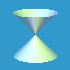x2+y2-z2=0 Quadratic cone.
45.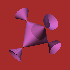x2+y2+z2+2x y z=1 Cubic with 4 singular points.
46.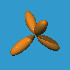x4+y4+z4+5x y z=0 A quartic surface with 4 branches touching at the origine.
47.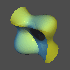x3+y3+z3=(x+y+z) a cubic surface with rotation.
48.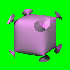x2 y2+y2 z2+z2 x2=(x2+y2+z2-0.75) a quartic surface.
49. cos(x)+cos(y)+cos(z) = 0 Schwarz Primitive Surface

It plots animation by clicking the spin checkbox.

### section3D for 4 graphes spin different directions

The section3D(x) combines 4 graphes into one plot,
surface: z=f(x,y) or f(x,y,z)=0
1. cut in from x-axis, spin around x-axis. 2. cut in from y-axis, spin around y-axis.
3. cross section, cut off from x-axis, spin around x-axis. 4. cross section, cut off from y-axis, spin around y-axis.

50. section3D
51. x*x
52. sin(x)
53. cos(x)
54. sin(x)-x
55. x-sin(x)
56. x**2+y**2=1
57. (x-2)**2-(y-2)**2

### implicit3D 隐函数图3D for equation f(x,y,z,t)=0

The implicit3D( ) can plot a 4-dimensional (x,y,z,t) object in 3D space. You manually change the t value by slider. e.g.
58. x*x-y*y-z*z-t-1 =0
59. 4*x^2 * (x^2 + y^2 + z^2 + z) + y^2 * (y^2 + z^2 - 1 - t) Lemniscate
More examples in Surface in 4D

### spin3D 旋转图3D for 4 graphes spin different directions

The spin3D( ) plots spin of implicit function for equation f(x,y)=0, and its inverse in 3D graph. It combines 4 graphes ( surface in 3D, space curve, plane curve and a curve spins to make stereo ) into one plot:
1. surface of equation f(x,y,z)=0, spin around x-axis. 2. spin around y-axis.
3. spin around z-axis. 4. positive value of a curve spins around z-axis to make stereo.
60. spin3D
61. x*x
62. sin(x)
63. cos(x)
64. sin(x)-x
65. x-sin(x)
66. x**2+y**2=1
67. (x-2)**2-(y-2)**2

### complex3D 复变函数图3D for 20 graphes in one plot

68. complex3D( ) plots a function on the complex plane and space, for 20 graphes in one plot.

In order to plot complex function, you should convert it to complex by tocomplex(x) before plot. Use complex(x) to set variable for complex variable in real value, and Use complex(0,x) to set variable for complex variable in imag value. Get real part by re() and imag part by im(). e.g. convert log(x) to re(log(complex(x))).

Here are some particularly nice examples by plot function complex3D( ) that show real and imaginary parts of complex function including special functions on complex axes and plane.

69. Bessel function of the first kind
70. Bessel function of the second kind
71. Hankel function of the first kind
72. Hankel function of the second kind
73. Jacobi elliptic sine
74. Jacobi elliptic cosine
75. Jacobi delta amplitude function
76. Gamma function
77. Riemann zeta function

More examples in complex function

#### the 4-dimensional object (x,y,z,t) in 3D space

78. the 4-dimensional object (x,y,z,t) in 3D space, manually change the t value by a slider
implicit3D(x*y*z-t)
More examples in Quadratic hypersurfaces in 4D

#### Animation

It plots animation by clicking the spin checkbox.
79.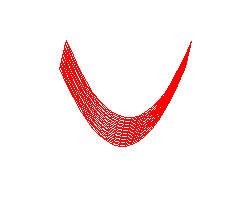Paraboloïd z=ax2+by2. One sees the surface deform with the variations of a and b.
80.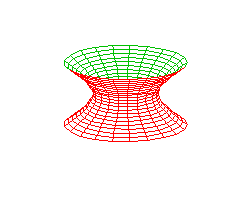Deformation of hyperboloid x2+y2+az2=1. Vertical.
81.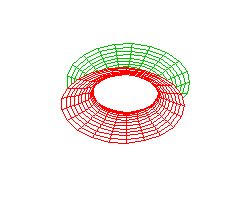Deformation of hyperboloïde x2+ay2+z2=1. Horizontal.
82.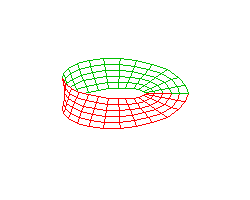The Moebius band.
83.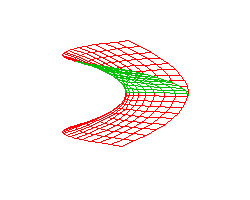Riemann surface of two sheets crossing each other.
84.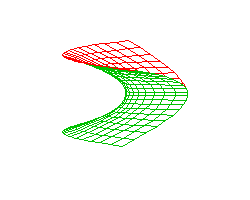A surface with a singular line, which resembles the precedent one but does not cross.
85.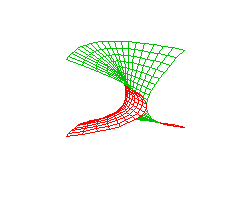Deformation of one into the other, for the above two surfaces. Without rotation.
86.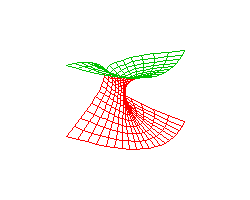As above but with rotation at the same time.
87.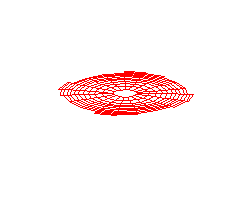A plane deforming into a cylinder then back to a plane from the other direction.
88.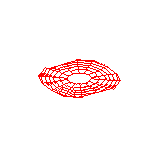A plane deforming into a cylinder then back to a plane from the other direction.
89. bianchi-pinkall-animation 花环
90. bianchi-pinkall-animation-zoomed 花环